# 4 Digit by 1 Digit Multiplication

Instructor: T.J. Hoogsteen

T.J. is currently a grade 5 teacher and Vice-Principal. He has a master's degree in Educational Administration and is working toward an Ed.D. in Educational Leadership.

In this lesson, you will learn how to do 4 digit by 1 digit multiplication. You will learn how to do these type of equations vertically and how to regroup.

## A Dream Trip

What is your dream vacation? Disney World? Universal Studios? Mine is Hawaii. Sun, sand and the beach. What could be better? I have 4 months until I can take a vacation and I need to start saving some money.

So I sit down to plan it out. I figure out that I can afford to save 1172 dollars each month. I have 4 months until my holidays, so I have that long to save.

If I am able to save 1172 dollars each month for 4 months, how much would I have? How can I figure it out?

Since I am going to save the same amount each month, I can multiply how much I am going to save by the number of times I would save that amount. So I figured out my equation would be 1172 x 4. When multiplying numbers that have more than one digit, you want to set your problem up vertically, with one number placed on top of the other. My savings equation looks like this: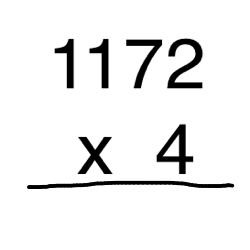There are two important things to remember when writing equations vertically: First, the number with the greater number of digits goes on top; Second, each of the numbers will line up in the ones column. Just look at our problem and you will see what I mean.

## 4-Digit Multiplication

Now the question is, how do I multiply an equation like this? To start, begin multiplying the digit in the ones place by the number in the bottom row (2 x 4).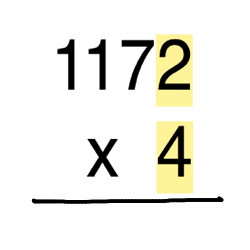When I multiply, the answer is 8. I place the 8 underneath the digits I just multiplied.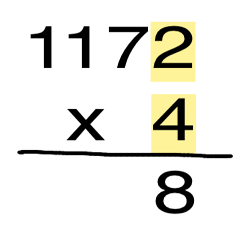Next, multiply the the digit in the tens column by the number in the bottom row (7 x 4).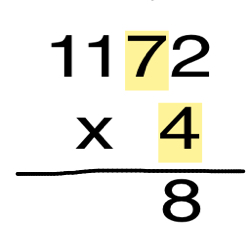When I multiply 7 x 4, the answer is 28. An important thing to remember is that only the 8 gets placed in the answer and the 2 gets regrouped to the next column, like this: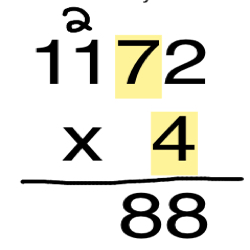I have to regroup in questions like this anytime the product, which is the answer you get when you multiply numbers, is 10 or greater. The next step is to multiply the number in the hundreds place by the number in the bottom row (1 x 4).To unlock this lesson you must be a Study.com Member.

### Register to view this lesson

Are you a student or a teacher?

#### See for yourself why 30 million people use Study.com

##### Become a Study.com member and start learning now.
Back
What teachers are saying about Study.com

### Earning College Credit

Did you know… We have over 200 college courses that prepare you to earn credit by exam that is accepted by over 1,500 colleges and universities. You can test out of the first two years of college and save thousands off your degree. Anyone can earn credit-by-exam regardless of age or education level.